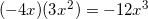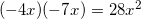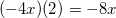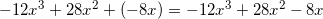High School Math : High School Math

Example Questions

← Previous 1 3 4 5 6 7 8 9 225 226

Example Question #1 : Distributive Property

Simplify the expression.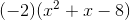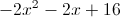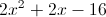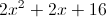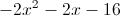Explanation:

Use the distributive property to multiply each term of the polynomial by. Be careful to distribute the negative as well.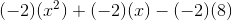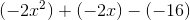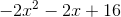Example Question #1 : Distributive Property

Find the value of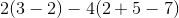.

4

6

-6

-2

2

2

Explanation:

We can seperate the problem into two steps: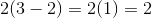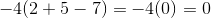We then combine the two parts: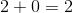Example Question #3 : Distributive Property

Distribute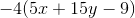.Explanation:

When distributing with negative numbers we must remember to distribute the negative to all of the variables in the parentheses.

Distribute thethrough the parentheses by multiplying it with each object in the parentheses to get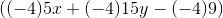.

Perform the multiplication remembering the positive/negative rules to get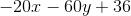, our answer.

Example Question #1 : Distributive Property

Simplify the expression.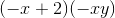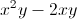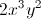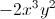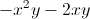Explanation:

Multiply the mononomial by each term in the binomial, using the distributive property.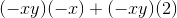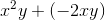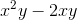Example Question #1 : High School Math

Simplify the expression.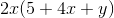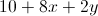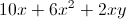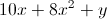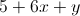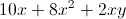Explanation:Use the distributive property to multiply each term by.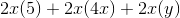Simplify.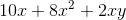Example Question #1 : High School Math

Simplify the following expression: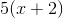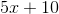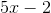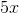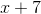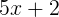Explanation:

Recall that the distributive property requires that we multiply the outside term by both terms in parentheses and add the results.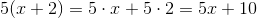Example Question #2 : High School Math

Evaluate the following expression: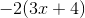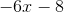None of the above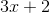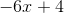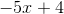Explanation:

Recall the distributive property. We need to multiply the outside factor by both terms inside and then combine.

Thus,Example Question #1 : Distributive Property

Distribute: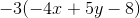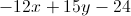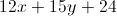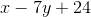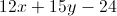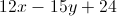Explanation:

When distributing with negative numbers we must remember to distribute the negative to all of the terms in the parentheses.

Remember, a negative multiplied by a negative is positive, and a negative multiplied by a positive number is negative.

Distribute thethrough the parentheses: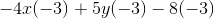Perform the multiplication, remembering the positive/negative rules: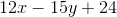Example Question #1 : Apply The Properties Of Operations To Generate Equivalent Expressions: Ccss.Math.Content.6.Ee.A.3

Which of the following is equivalent to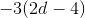?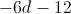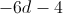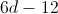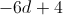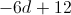Explanation:

We need to distribute -3 by multiplying both terms inside the parentheses by -3.:.

Now we can multiply and simplify. Remember that multiplying two negative numbers results in a positive number: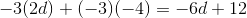Example Question #2 : High School Math

Expand: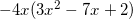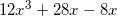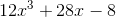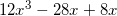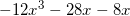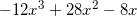Explanation:Use the distributive property. Do not forget that the negative sign needs to be distributed as well!